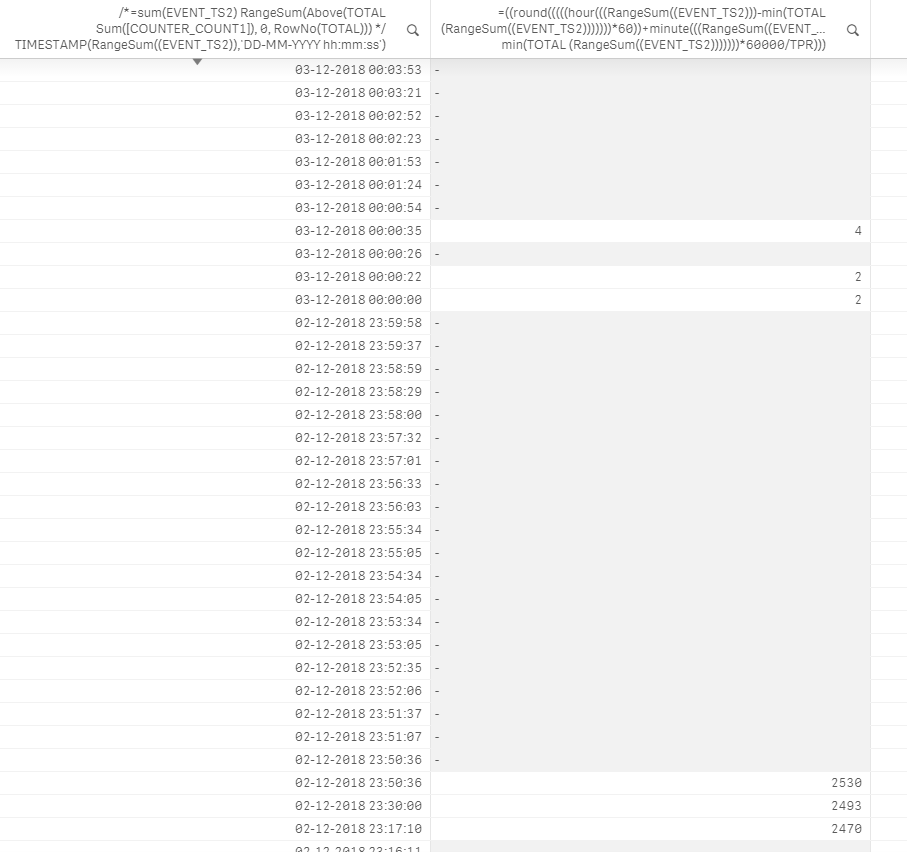# Qlik Sense App Development

Announcements
Uploads getting stuck in the virus scanner. We are investigating.
cancel
Showing results for
Did you mean:
HighlightedContributor II

## Correct script(date calculation)

Hi fellos,

Can somebody have idea why my calculation stop counting  and reset calculation based on 24 hours?((round(((((hour(((RangeSum((EVENT_TS2)))-min(TOTAL (RangeSum((EVENT_TS2)))))))*60))+minute(((RangeSum((EVENT_TS2)))-min(TOTAL (RangeSum((EVENT_TS2)))))))*60000/TPR))))

Labels (1)
• ### Qlik Community